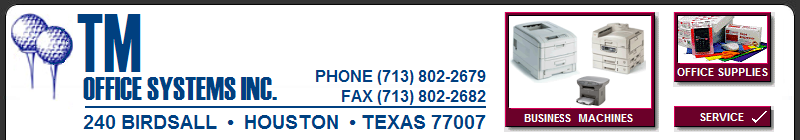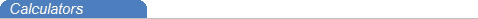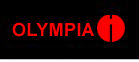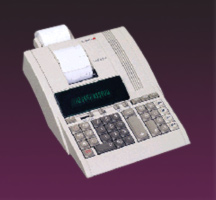BUSINESS MACHINESPrinters
 HP Lexmark OkiData
Copiers
FAXs
Multi-function
 Lexmark OkiData
Calculators
Shredders
 Fellows FORMAX GBC MARTINYALECPD5612CPD5712 CPD5714Percent change key calculates the percentage increase or decrease between two numbers. - Backspace key allows correction of single digit in series. - Markup calculates selling price and gross profit. - 4-key independent memory adds or subtracts results in memory register without affecting the current calculation item count counts plus and minus operations.Delta percent change key calculates the percentage increase or decrease between two numbers - Backspace key allows correction of single digit in series - Fixed decimal selection: A,F,0,2,3,4,6 - Algebraic change key changes the algebraic sign of a number in the keyboard register - Markup calculates selling price and gross profit - 4-key independent memory.Delta percent change key calculates the percentage increase or decrease between two numbers - Backspace key allows correction of single digit in series - Fixed decimal selection: A,F,0,2,3,4,6 - Algebraic change key changes the algebraic sign of a number in the keyboard register - Markup calculates selling price and gross profit. independent memory. Constant to memory retains the multiplicand and divisor for use with the 4-key independent memory CALL CALL CALL